# 李航-统计学习方法-笔记-7：支持向量机

## 线性可分SVM

$\hat{\gamma_i} = y_i (w \cdot x_i + b)$

$\hat{\gamma} = \min_{i=1, ..., N} \hat{\gamma_i}$

$\gamma_i = y_i (\frac{w}{||w||} \cdot x_i + \frac{b}{||w||})$

$\gamma = \min_{i=1, ..., N} \gamma_i$

$\max_{w,b} \ \gamma$

$s.t. \ \ y_i (\frac{w}{||w||} \cdot x_i + \frac{b}{||w||}) \geqslant \gamma, \ \ i = 1, 2, ..., N$

$\max_{w,b} \ \frac{\hat{\gamma}}{||w||}$

$s.t. \ \ y_i (w \cdot x_i + b) \geqslant \hat{\gamma}, \ \ i = 1, 2, ..., N$

$\min_{w, b} \frac{1}{2} || w || ^2, \tag{7.13}$

$s.t. \ \ y_i ( w \cdot x_i + b) - 1 \geqslant 0, \ \ i=1, 2, ..., N \tag{7.14}$

$w^* \cdot x + b^* = 0 $$以及分类决策函数$$f(x) = sign(w^* \cdot x + b^*)$，即线性可分SVM。

$\min_w \ \ f(w)$

$\begin{split} s.t. \ \ &g_i(w) \leqslant 0, i=1, 2, ..., k \\ &h_i(w) = 0, i=1, 2, ..., l \end{split}$

$$h(x)$$称为仿射函数，如果它满足$$h(x) = a \cdot x + b$$$$a \in R^n, b \in R, x \in R^n$$

$y_i (w \cdot x_i + b) - 1 = 0$

$$y_i = +1$$的正例点，支持向量在超平面$$H_1: w \cdot x + b = +1$$
$$y_i = -1$$的正例点，支持向量在超平面$$H_2: w \cdot x + b = -1$$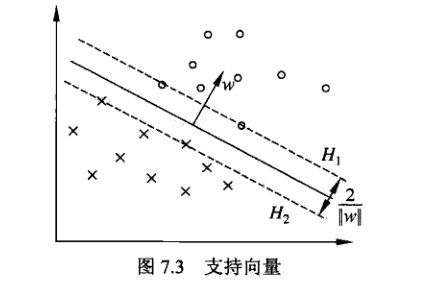## 求解线性可分SVM

$L(w, b, \alpha) = \frac{1}{2}||w||^2 - \sum_{i=1}^{N}\alpha_i y_i (w \cdot x_i + b) + \sum_{i=1}^{N}\alpha_i \tag{7.18}$

（1）先求$$\min_{w,b} L(w, b, \alpha)$$，分别对$$w,b$$求偏导，令其等于0。

$\nabla_w L(w, b, \alpha) = w - \sum_{i=1}^{N}\alpha_i y_i x_i = 0$

$\nabla_b L(w, b, \alpha) = -\sum_{i=1}^{N}\alpha_i y_i = 0$

$w = \sum_{i=1}^{N}\alpha_i y_i x_i, \tag{7.19}$

$\sum_{i=1}^{N}\alpha_i y_i = 0, \tag{7.20}$

$\begin{split} &\min_{w, b} L(w, b, \alpha) \\ &= \frac{1}{2} \sum_{i=1}^{N} \sum_{j=1}^{N} \alpha_i \alpha_j y_i y_j(x_i \cdot x_j) - \sum_{i=1}^{N} \alpha_i y_i [(\sum_{j=1}^{N} \alpha_j y_j x_j) \cdot x_i + b] + \sum_{i=1}^{N} \alpha_i \\ &= - \frac{1}{2} \sum_{i=1}^{N} \sum_{j=1}^{N} \alpha_i \alpha_j y_i y_j(x_i \cdot x_j) + \sum_{i=1}^{N} \alpha_i \end{split}$

（2）求$$\min_{w, b} L(w, b, \alpha)$$$$\alpha$$的极大，即是对偶问题

$\max_\alpha - \frac{1}{2} \sum_{i=1}^{N} \sum_{j=1}^{N} \alpha_i \alpha_j y_i y_j(x_i \cdot x_j) + \sum_{i=1}^{N} \alpha_i, \tag{7.21}$

$s.t. \ \ \sum_{i=1}^{N}\alpha_i y_i = 0$

$\alpha_i \geqslant 0, \ \ i = 1,2, ..., N$

$\max_\alpha - \frac{1}{2} \sum_{i=1}^{N} \sum_{j=1}^{N} \alpha_i \alpha_j y_i y_j(x_i \cdot x_j) + \sum_{i=1}^{N} \alpha_i \tag{7.22}$

$s.t. \ \ \sum_{i=1}^{N}\alpha_i y_i = 0 \tag{7.23}$

$\alpha_i \geqslant 0, \ \ i = 1,2, ..., N \tag{7.24}$

$w^* = \sum_{i}\alpha_i^* y_i x_i \tag{7.25}$

$b^* = y_j - \sum_{i=1}^{N} \alpha_i^* y_i (x_i \cdot x_j) \tag{7.26}$

$\nabla_w L(w^*, b^*, \alpha^*) = w^* - \sum_{i=1}^{N}\alpha_i^* y_i x_i = 0 \tag{7.27}$

$\nabla_b L(w^*, b^*, \alpha^*) = -\sum_{i=1}^{N}\alpha_i^* y_i = 0$

$\alpha_i^* (y_i ( w^* \cdot x_i + b^*) - 1) = 0, \ \ i=1, 2, ..., N$

$y_i ( w^* \cdot x_i + b^*) - 1 \geqslant 0, \ \ i=1, 2, ..., N$

$\alpha^* \geqslant 0, i=1, 2, ..., N$

$w^* = \sum_{i}\alpha_i^* y_i x_i$

$y_j (w^* \cdot x_j + b^*) - 1 = 0 \tag{7.28}$

$b^* = y_j - \sum_{i=1}^{N} \alpha_i^* y_i (x_i \cdot x_j)$

$\sum_{i=1}^{N} \alpha_i^* y_i (x \cdot x_i) + b^* = 0 \tag{7.29}$

$f(x) = sign(\sum_{i=1}^{N} \alpha_i^* y_i (x \cdot x_i) + b^*) \tag{7.30}$

$\alpha_i^* (y_i ( w^* \cdot x_i + b^*) - 1) = 0, \ \ i=1, 2, ..., N$

$w^* \cdot x_i + b^* = \pm1$

$$x_i$$一定在间隔边界上，符合前面支持向量的定义。

## 线性SVM和软间隔最大化

$y_i(w \cdot x_i + b) \geqslant 1 - \xi_i$

$\frac{1}{2} || w ||^2 + C \sum_{i=1}^{N} \xi_i \tag{7.31}$

$\min_{w, b, \xi} \frac{1}{2} || w || ^2 + C \sum_{i=1}^{N} \xi_i \tag{7.32}$

$s.t. \ \ y_i ( w \cdot x_i + b) \geqslant 1 - \xi_i, \ \ i=1, 2, ..., N \tag{7.33}$

$\xi_i \geqslant 0, \ \ i=1, 2, ..., N \tag{7.34}$

## 求解线性SVM

$\begin{split} L(w, b, \xi, \alpha, \mu) &= \frac{1}{2}||w||^2 + C \sum_{i=1}^{N} \xi_i - \sum_{i=1}^{N}\mu_i \xi_i \\ &- \sum_{i=1}^{N}\alpha_i (y_i (w \cdot x_i + b) -1 + \xi_i) \end{split} \tag{7.40}$

$\nabla_w L(w, b, \xi, \alpha, \mu) = w - \sum_{i=1}^{N}\alpha_i y_i x_i = 0 \tag{7.41}$

$\nabla_b L(w, b, \xi, \alpha, \mu) = -\sum_{i=1}^{N}\alpha_i y_i = 0 \tag{7.42}$

$\nabla_\xi L(w, b, \xi, \alpha, \mu) = C - \alpha_i - \mu_i = 0 \tag{7.43}$

$\min_{w, b, \xi} L(w, b, \xi, \alpha, \mu) = - \frac{1}{2} \sum_{i=1}^{N} \sum_{j=1}^{N} \alpha_i \alpha_j y_i y_j(x_i \cdot x_j) + \sum_{i=1}^{N} \alpha_i$

$\max_\alpha - \frac{1}{2} \sum_{i=1}^{N} \sum_{j=1}^{N} \alpha_i \alpha_j y_i y_j(x_i \cdot x_j) + \sum_{i=1}^{N} \alpha_i \tag{7.44}$

$s.t. \ \ \sum_{i=1}^{N} \alpha_i y_i = 0 \tag{7.45}$

$C - \alpha_i - \mu_i = 0 \tag{7.46}$

$\alpha_i \geqslant 0 \tag{7.47}$

$\mu_i \geqslant 0 \ \ i=1,2, ..., N \tag{7.48}$

$0 \geqslant \alpha_i \geqslant C \tag{7.49}$

$\min_\alpha \frac{1}{2} \sum_{i=1}^{N} \sum_{j=1}^{N} \alpha_i \alpha_j y_i y_j(x_i \cdot x_j) - \sum_{i=1}^{N} \alpha_i \tag{7.37}$

$s.t. \ \ \sum_{i=1}^{N} \alpha_i y_i = 0 \tag{7.38}$

$0 \geqslant \alpha_i \geqslant C, \ \ i=1,2, ..., N \tag{7.39}$

$w^* = \sum_{i=1}^N \alpha_i^* y_i x_i \tag{7.50}$

$b^* = y_j -\sum_{i=1}^N y_i \alpha_i^*(x_i \cdot x_j) \tag{7.51}$

$\nabla_w L(w^*, b^*, \xi^*, \alpha^*, \mu^*) = w^* - \sum_{i=1}^{N}\alpha_i^* y_i x_i = 0 \tag{7.52}$

$\nabla_b L(w^*, b^*, \xi^*, \alpha^*, \mu^*) = -\sum_{i=1}^{N}\alpha_i^* y_i = 0$

$\nabla_\xi L(w^*, b^*, \xi^*, \alpha^*, \mu^*) = C - \alpha_i^* - \mu_i^* = 0$

$\alpha_i^*(y_i(w^* \cdot x_i + b^*) - 1 + \xi_i^*) = 0 \tag{7.53}$

$\mu_i^* \xi_i^* = 0 \tag{7.54}$

$y_i(w^* \cdot x_i + b^*) - 1 + \xi_i^* \geqslant 0$

$\xi_i^* \geqslant 0$

$\alpha_i^* \geqslant 0$

$\mu_i^* \geqslant 0, \ \ i=1, 2, ..., N$

$\sum_{i=1}^N \alpha_i^* y_i (x \cdot x_i) + b^* = 0 \tag{7.55}$

$f(x) = sign(\sum_{i=1}^N \alpha_i^* y_i (x \cdot x_i) + b^*) \tag{7.56}$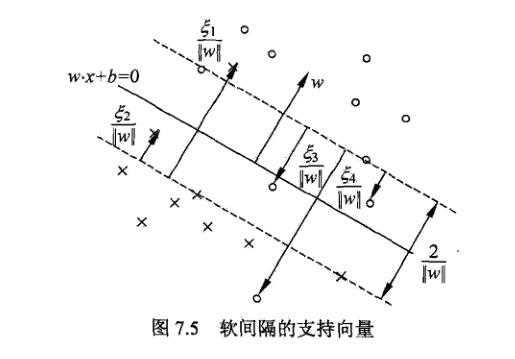$$\alpha_i^* < C$$，则$$\xi_i = 0$$，支持向量$$x_i$$恰好落在间隔边界上。
$$\alpha_i^* = C, \ 0 < \xi_i < 1$$，则分类正确，$$x_i$$在间隔边界与分离超平面之间。
$$\alpha_i^* = C, \xi_i = 1$$，则分类正确，$$x_i$$在间隔边界与分离超平面之间。
$$\alpha_i^* = C, \xi_i > 1$$，则$$x_i$$位于分离超平面误分一侧。

## 合页损失函数

$\sum_{i=1}^{N}[1 - y_i (w \cdot x_i + b)]_+ + \lambda||w||^2 \tag{7.57}$

$L(y(w \cdot x + b)) = [1 - y(w \cdot x + b)]_+ \tag{7.58}$

$[z]_+ = \left\{\begin{matrix} z, & z>0 \\ 0, & z\leqslant0 \end{matrix}\right. \tag{7.59}$

$\min_{w, b, \xi} \frac{1}{2} || w || ^2 + C \sum_{i=1}^{N} \xi_i \tag{7.60}$

$s.t. \ \ y_i ( w \cdot x_i + b) \geqslant 1 - \xi_i, \ \ i=1, 2, ..., N \tag{7.61}$

$\xi_i \geqslant 0, \ \ i=1, 2, ..., N \tag{7.62}$

$\min_{w,b} \sum_{i=1}^{N}[1 - y_i (w \cdot x_i + b)]_+ + \lambda||w||^2 \tag{7.63}$

$[1 - y_i (w \cdot x_i + b)]_+ = \xi_i \tag{7.64}$

$$\xi_i \geqslant 0$$，式$$(7.62)$$成立。由式$$(7.64)$$

$$1 - y_i (w \cdot x_i + b) > 0$$时，有$$y_i (w \cdot x_i + b) = 1 - \xi_i$$
$$1 - y_i (w \cdot x_i + b) \leqslant 0$$时，有$$y_i (w \cdot x_i + b) \geqslant 1 - \xi_i$$

$\min_{w,b} \sum_{i=1}^N \xi_i + \lambda ||w||^2$

$\min_{w,b} \frac{1}{C} (\frac{1}{2}||w||^2 + C \sum_{i=1}^N \xi_i)$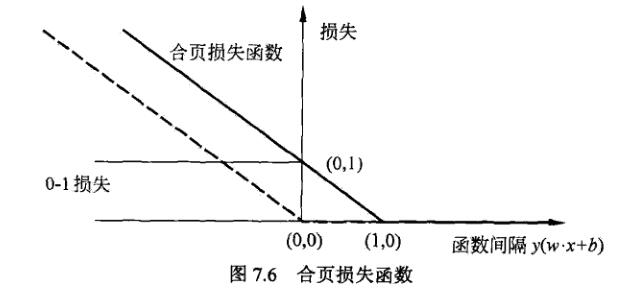### 非线性SVM与核函数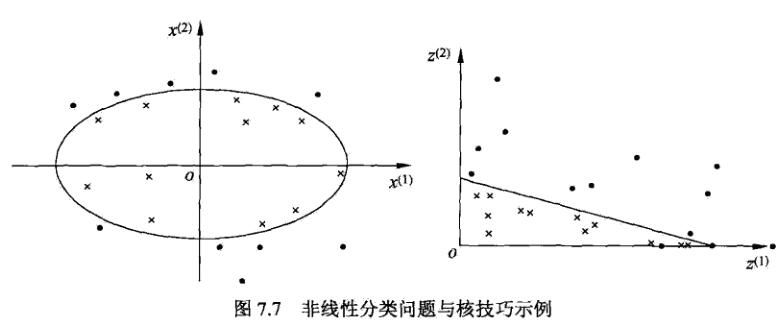$z = \phi(x) = ((x^{(1)})^2, (x^{(2)})^2)^T$

$$\mathcal{X}$$是输入空间，又设$$\mathcal{H}$$为特征空间，如果存在一个从$$\mathcal{X}$$$$\mathcal{H}$$的映射$$\phi(x): \mathcal{X} \to \mathcal{H}$$，使得对所有$$x, z \in \mathcal{X}$$，函数$$K(x, z)$$满足条件：

$K(x, z) = \phi(x) \cdot \phi(z)$

$W(\alpha) = \frac{1}{2} \sum_{i=1}^{N} \sum_{j=1}^{N} \alpha_i \alpha_j y_i y_j K(x_i, x_j) - \sum_{i=1}^{N} \alpha_i$

$f(x) = sign(\sum_{i=1}^N \alpha_i^* y_i K(x, x_i) + b^*)$

$$K: \mathcal{X} \times \mathcal{X} \to R$$是对称函数，则$$K(x, z)$$为正定核函数的充要条件是对任意$$x_i \in \mathcal{X}, \ \ i=1, 2, ...,m$$$$K(x, z)$$对应的Gram矩阵：

$K = [K(x_i, x_j)]_{mxm}$

（1）多项式核函数（polynomial kernel function）

$K(x, z) = (x \cdot z + 1)^p$

$f(x) = sign(\sum_{i=1}^{N_s} \alpha_i^* y_i (x_i \cdot x + 1)^p + b^*)$

（2）高斯核函数（Gaussian kernel function）

$K(x, z) = exp(- \frac{||x-z||^2}{2\sigma^2})$

$f(x) = sign(\sum_{i=1}^{N_s} \alpha_i^* y_i \ exp(- \frac{||x-z||^2}{2\sigma^2}) + b^*)$

（3）字符串核函数

（1）给定一个核函数$$K$$，对于$$a>0$$$$aK$$也为核函数。
（2）给定两个核函数$$K'$$$$K''$$$$K' K''$$也为核函数。
（3）对输入空间的任意实值函数$$f(x)$$$$K(x_1, x_2) = f(x_1) f(x_2)$$为核函数。

### 序列最小最优化算法

SVM的学习问题可以形式化为求解凸二次规划问题。这样的凸二次规划问题具有全局最优解，并且有许多最优化算法可以用于这一问题的求解。但是当训练样本容易很大时，这些算法往往变得非常低效，以致无法使用。如何高效地实现SVM就成为一个重要问题。

SMO（sequential minimal optimization）是其中一种快速学习算法。解如下凸二次规划的对偶问题

$\min_\alpha \frac{1}{2} \sum_{i=1}^{N} \sum_{j=1}^{N} \alpha_i \alpha_j y_i y_j K(x_i, x_j) - \sum_{i=1}^{N} \alpha_i \tag{7.98}$

$s.t. \ \ \sum_{i=1}^{N} \alpha_i y_i = 0 \tag{7.99}$

$0 \geqslant \alpha_i \geqslant C, \ \ i=1,2, ..., N \tag{7.100}$

SMO算法：SMO算法是一种启发式算法，基本思路是：如果所有变量的解都满足此最优化问题的KKT条件，那么解就得到了。否则，选择两个变量，固定其它变量，针对这两个变量构建一个二次规划问题。这个二次规划问题关于这两个变量的解应该更接近原始二次规划问题的解，因为这会使得原始二次规划问题的目标函数变得更小。重要的是，这时子问题可以通过解析方法求解，就可以大大提高计算速度。

$\min_{\alpha_1, \alpha_2} W(\alpha_1, \alpha_2) = \frac{1}{2} K_{11} \alpha_1^2 + \frac{1}{2} K_{22} \alpha_2^2 + \\ y_1 y_2 K_{12} \alpha_1 \alpha_2 - (\alpha_1 + \alpha_2) + y_1 \alpha_1 \sum_{i=3}^N y_i \alpha_i K_{i1} + y_2 \alpha_2 \sum_{i=3}^N y_i \alpha_i K_{i2} \tag{7.101}$

$s.t. \ \ \alpha_1 y_1 + \alpha_2 y_2 = -\sum_{i=3}^N y_i \alpha_i = \zeta \tag{7.102}$

$0 \leqslant \alpha_i \leqslant C, \ \ i=1,2 \tag{7.103}$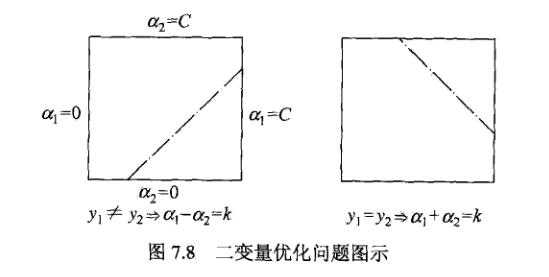$L \leqslant \alpha_2^{new} \leqslant H$

$L = \max(0, \alpha_2^{old} - \alpha_1^{old}), \ \ H = \min(C, C+\alpha_2^{old} - \alpha_1^{old})$

$L = \max(0, \alpha_2^{old} + \alpha_1^{old} - C), \ \ H = \min(C, \alpha_2^{old} + \alpha_1^{old})$

$g(x) = \sum_{i=1}^N \alpha_i y_i K(x_i, x) + b$

$E_i = g(x_i) - y_i = \sum_{j=1}^N \alpha_j y_j K(x_j, x_i) + b - y_i$

$$i=1,2$$时，$$E_i$$为函数$$g(x)$$对输入$$x_i$$的预测值与真实输出$$y_i$$之差。

$\alpha_2^{new, unc} = \alpha_2^{old} + \frac{y_2(E_1 - E_2)}{\eta}$

$\eta = K_{11} + K_{22} - 2K_{12} = ||\phi(x_1) - \phi(x_2)||^2$

$\alpha_2^{new} = \left\{\begin{matrix} H, & \alpha_2^{new, unc}> H \\ \alpha_2^{new, unc}, & L \leqslant \alpha_2^{new, unc} \leqslant H \\ L, & \alpha_2^{new, unc} < L \end{matrix}\right.$

$$\alpha_2^{new}$$求得$$\alpha_1^{new}$$

$\alpha_1^{new} = \alpha_1^{old} + y_1 y_2 (\alpha_2^{old} - \alpha_2^{new})$

$v_i = \sum_{j=3}^N \alpha_i y_i K(x_i, x_j) = g(x_i) - \sum_{j=1}^2 \alpha_i y_i K(x_i, x_j) - b$

$W(\alpha_1, \alpha_2) = \frac{1}{2} K_{11} \alpha_1^2 + \frac{1}{2} K_{22} \alpha_2^2 + y_1 y_2 K_{12} \alpha_1 \alpha_2 \\ - (\alpha_1 + \alpha_2) + y_1 v_1 \alpha_1 + y_2 v_2 \alpha_2 \tag{7.110}$

$$\alpha_1 y_1 = \zeta - \alpha_2 y_2$$$$y_j^2 = 1$$，可将$$\alpha_1$$表示为

$\alpha_1 = (\zeta - y_2 \alpha_2) y_1$

$W(\alpha_2) = \frac{1}{2} K_{11} (\zeta - \alpha_2 y_2)^2 + \frac{1}{2} K_{22} \alpha_2^2 + y_2 K_{12} (\zeta - \alpha_2 y_2) \alpha_2 \\ - (\zeta - y_2 \alpha_2) y_1 - \alpha_2 + y_1 v_1 (\zeta - y_2 \alpha_2) y_1 + y_2 v_2 \alpha_2$

$$\alpha_2$$求导数

$\frac{\partial W}{\partial \alpha_2}=K_{11} \alpha_2 + K_{22} \alpha_2 - 2 K_{12} \alpha_2 - K_{11} \zeta y_2 + K_{12} \\+ y_1 y_2 - 1 - v_1 y_2 + y_2 v_2$

$\alpha_2^{new, unc} = \alpha_2^{old} + \frac{y_2(E_1 - E_2)}{\eta}$

SMO算法在每个子问题中选择两个变量优化，其中至少一个变量是违反KKT条件的。
（1）第一个变量的选择
SMO称选择第1个变量的过程为外层循环。外层循环在训练样本中选取违反KKT条件最严重的样本，并将其对应的变量作为第1个变量。具体地，检查训练样本点是否满足KKT条件，即

$\alpha_i = 0 \Leftrightarrow y_i g(x_i) \geqslant 1 \\ 0 < \alpha_i < C \Leftrightarrow y_i g(x_i) = 1 \\ \alpha_i = C \Leftrightarrow y_i g(x_i) \leqslant 1$

（2）第二个变量的选择
SMO称选择第2个变量的过程为内部循环。选取的标准是希望$$\alpha_2$$有足够大的变化。由于$$\alpha_2^{new}$$是依赖于$$|E_1 - E_2|$$的，为了加快计算速度，一种简单的做法是选择$$\alpha_2$$，使其对应的$$|E_1 - E_2|$$最大。因为$$\alpha_1$$已定，$$E_1$$也确定了。如果$$E_1$$为正，那么选择最小的$$E_i$$作为$$E_2$$；如果$$E_1$$为负，那么选择最大的$$E_i$$作为$$E_2$$。为了节省计算事件，将所有$$E_i$$值保存在一个列表中。

（3）计算阈值$$b$$和差值$$E_i$$

posted @ 2019-06-05 15:51  PilgrimHui  阅读(2351)  评论(2编辑  收藏  举报